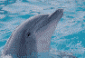# MxConstraint Problem

5 posts / 0 newOffline
Joined: 10/19/2009 - 15:38
MxConstraint Problem

(1) ran three models: the first a "general model", the second and third models constrained a (1 x 4) vector of means for one group to equal the (1 x 4) vector of means for another group. the second model did this using an MxAlgebra object while the third used an MxConstraint object.
(2) all models converged with an NPSOL inform value of 0.
(3) gradient and hessian were perfectly fine for models 1 and 2 but not for model 3. largest gradient element was -40 and the norm of the gradient was 5900. the search direction at convergence (inverse(hess) %*% gradient) gave very large elements. the calculated hessian was a (1 x 1) matrix with element NA.
(4) yet, the second and third models gave the same function value and the same parameter estimates.
(5) suspect something funky with the gradient and/or hessian returned with the third model.

belatedly, noticed that i could not attach a *.zip file. go to

gregOffline
Joined: 07/31/2009 - 15:12
The hessian returned in he

The hessian returned in he third model is not the full hessian, but in fact a submatrix of a hessian that includes both the parameters and the constraints. Standard errors aren't likely to be valid when mxConstraint objects are in a model, as they are based on his partial hessian. As you noted, parameter estimates and fit are equivalent regardless of how a constraint is made. We try to encourage users to avoid mxConstraint for equality whenever possible; it is best used only for non-linear constraints involving inequalities.

ryneOffline
Joined: 07/31/2009 - 15:14
Or equality constraints for

Or equality constraints for which there isn't a simple direct algebraic solution - G-E covariance in the presence of genetic & cultural transmission and assortative mating is one example.

Indeed, I think that that the returned approximate ("estimated") hessian in the presence of non-linear constraints is incorrect, and if possible we should fix it. The calculated Hessian is not available because its algorithm doesn't understand constraints either.Offline
Joined: 10/19/2009 - 15:38
thanks all, m familiar with

thanks all,

m familiar with these issues about constraints. my purpose was to point out a potential problem with NPSOL and/or OpenMx.

the gradient issue is the most important one. the constraints should NOT influence the gradient's calculation, so getting numbers like 40 instead of .0001 implies an error somewhere.

re the estimated hessian. ask: if this is wrong and the gradient is wrong, then how does NPSOL ever find the correct solution? i rule out divine intervention.Offline
Joined: 07/31/2009 - 15:14
Agreed, the problem appears

Agreed, the problem appears to be cosmetic - what is reported by OpenMx is incorrect, whereas what is internal to NPSOL is evidently not.